# Ring (mathematics)

This article or section needs to be written in plain English, using plain English that most of our readers can understand. Articles that depend excessively on technical terms accessible only to specialists are useless for our purposes, so writers are admonished to avoid jargon

A ring in mathematics is a set R equipped with two binary operations, usually called addition and multiplication, that is a group under the operation of addition and a monoid (no inverses) under the operation of multiplication.

## Examples

•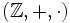- the set of the integers - together with the usual addition and multiplication is a ring.
• For any ringthe polynomial ring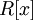consisting of elements of the form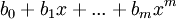where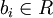is a ring as well. All polynomials with integer coefficients form the ring.
•: this is the ring of six elements {0,1,2,3,4,5} and the usual addition and multiplication modulo six. So, here 1+3= 4, but 4+5 = 3. Interestingly,, so, you can multiply two elements, neither of which is zero, and get zero as the result! This means that this ring is not an domain.
•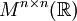, the set of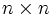real matrices, with operations of matrix addition and multiplication, is a ring.

## Axioms

Explicitly, a ringis a set, with two binary operations usually labeledand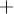that satisfy the following axioms.

1. Identity - there exists an element (usually labeled) such that2. Associativity - for any three elements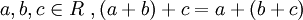3. Inverses - for any elementthere exists an element (usually labeled assuch that)
4. 'Commutativity - for any two elements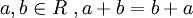Axioms of Multiplication
1. Identity* - there exists an element (usually labeled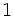) such that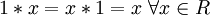2. Associativity - for any three elements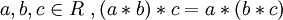Distributive Property
1. For three elements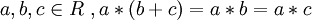* - Some texts define a ring without a multiplicative identity. See rng

## Elements

An element of a ringis a unit if it has a multiplicative inverse. The numbersare the only units in the Integers

An element of ais a zero divisor if there is another element. In the third example above, 2 and 3 are zero divisors. In fact, for any ring of Integers modulo m, all prime factors of m and their producs are zero divisors.

The multiplicative identity (usually denoted as) is the unique element of the ring thatThe additive Identity (usually denoted as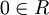) is the unique element of the ring thatA prime element (or prime) is an element of a commutative ring s.t. a non-unitis prime if and only if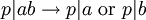(note that this differs from the definition of a prime that is more commonly presented in grade school).

An irreducible element is an element of an integral domain (a special type of ring) such thatis irreducible if it cannot be written as a product of two non-units. When applied to the integers (which is an integral domain) this means that an irreducible element is any element that only has itself and 1 as prime factors (1 is unit, so we satisfy the definition), this is the common way to define prime numbers. Note that every prime element is necessarily irreducible, but not every irreducible element is necessarily a prime element (this motivates us to exclude 1 from the list of prime numbers)

## Remarks

A rng (pronounced "rung") is an a ring without a multiplicative identity. In some texts, a ring is defined as rng, it turns out that many of the most elementary theorems (the ones that apply to arbitrary rings) do not require an identity, this ambiguity causes the confusion.

In texts where a ring is defined as a rng - a ring with unity is a rng with a multiplicative identity (our definition of a ring)

A commutative ring is a ring in which multiplication is commutative. The first three examples of rings given above are commutative rings: the last is not, since matrix multiplication is not in general commutative. The study of the properties of commutative rings is usually called commutative algebra.

An entire ring is a ring that has no zero divisors.

An integral domain is a commutative entire ring.

A Unique Factorization Domain (UFD) is an integral domain in which every element that is not 0 or a unit can be written as a product of irreducible elements. i.e. every element can be factored into a product of primes, analogous to the integers (In a related theorem, every irreducible element of a UFD is prime)

A Field is a commutative ring where every element except 0 is a unit (is invertible). In other words, a field is a ring that is an abelian group over both addition and multiplication on the non-zero elements.

A division ring (also known as a skew field) is a ring that is a group over multiplication (every non-zero element has an inverse, but not necessarily commutative). A division ring can also be thought of as a field that is not commutative.